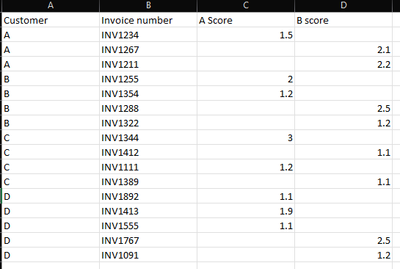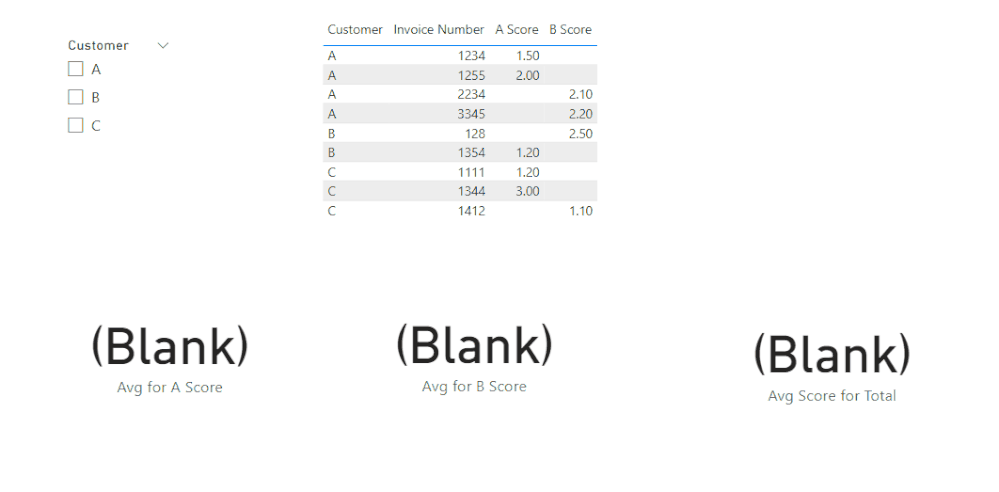cancel
Showing results for
Did you mean:Frequent Visitor

## How do i compute average score

Hi guys!

Need help on this.I am trying to create a customer dashboard based on scoring of each customer. So when i filter to customer A for example, it will show a simple average of A score, B score and total of A and B score on card visuals. May i know what kind of measures or dax will make it possible?

Thanks!

1 ACCEPTED SOLUTIONCommunity Support

Hi @Sodared123 ,

1. below is my test table

Table:2. create three measure with below dax formula

``````Avg for A Score =
VAR cur_ct =
SELECTEDVALUE ( 'Table'[Customer] )
VAR tmp =
FILTER ( ALL ( 'Table' ), 'Table'[Customer] = cur_ct )
RETURN
AVERAGEX ( tmp, [A Score] )
``````
``````Avg for B Score =
VAR cur_ct =
SELECTEDVALUE ( 'Table'[Customer] )
VAR tmp =
FILTER ( ALL ( 'Table' ), 'Table'[Customer] = cur_ct )
RETURN
AVERAGEX ( tmp, [B Score] )
``````
``Avg Score for Total = [Avg for A Score]+[Avg for B Score]``

3. add a slicer with "Table[Customer]" column, add three card visual with above measure, add a table visual with fieldsPlease refer the attached .pbix file.

Best regards,
Community Support Team_ Binbin Yu
If this post helps, then please consider Accept it as the solution to help the other members find it more quickly.

4 REPLIES 4Community Support

Hi @Sodared123 ,

1. below is my test table

Table:2. create three measure with below dax formula

``````Avg for A Score =
VAR cur_ct =
SELECTEDVALUE ( 'Table'[Customer] )
VAR tmp =
FILTER ( ALL ( 'Table' ), 'Table'[Customer] = cur_ct )
RETURN
AVERAGEX ( tmp, [A Score] )
``````
``````Avg for B Score =
VAR cur_ct =
SELECTEDVALUE ( 'Table'[Customer] )
VAR tmp =
FILTER ( ALL ( 'Table' ), 'Table'[Customer] = cur_ct )
RETURN
AVERAGEX ( tmp, [B Score] )
``````
``Avg Score for Total = [Avg for A Score]+[Avg for B Score]``

3. add a slicer with "Table[Customer]" column, add three card visual with above measure, add a table visual with fieldsPlease refer the attached .pbix file.

Best regards,
Community Support Team_ Binbin Yu
If this post helps, then please consider Accept it as the solution to help the other members find it more quickly.Frequent Visitor

Hello guys! Any kind souls?Frequent Visitor

Hi Amit

Not sure if you get my requirements right, based on below table date, if i filter for customer A, i will see 1.75 (average of 1.5 and 2) in A score card, 2.15 (based on average of 2.1 and 2.2) in B score card, and 3.9 in the total score card. In this case, how many measures should i be creating and what will the DAX look like? Thanks alot!

 Customer Invoice Number A Score B Score A 1234 1.5 A 2234 2.1 A 3345 1.6 A 1255 2 B 1354 1.2 B 1288 2.5 C 1344 3 C 1412 1.1 C 1111 1.2Super User

@Sodared123 , Try like

Averagex(Summarize(Table, Table[Customer], Table[Invoice Number]), calculate(Sum(Table[A Score]) + Sum(Table[A Score]) ) )

If this does not help
Can you share sample data and sample output in table format? Or a sample pbix after removing sensitive data.